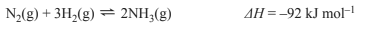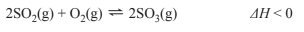Equilibrium (2017 VCE) 1) Ammonia, NH3, can be produced by the reaction of hydrogen, H2, and nitrogen, N2. When this reaction takes place in a sealed container of fixed volume, an equilibrium system is established. The equation for the reaction is shown below.If the pressure and volume remain constant when the temperature is increased, the forward reaction rate will A. increase and the [NH3] will increase. B. increase and the [NH3] will decrease. C. decrease and the [NH3] will decrease. D. decrease and the [NH3] will remain the same. Solution2) Sulfur trioxide, SO3, is made by the reaction of sulfur dioxide, SO2, and oxygen, O2, in the presence of a catalyst, according to the equation below.In a closed system in the presence of the catalyst, the reaction quickly achieves equilibrium at 1000 K. a. A mixture of 2.00 mol of SO2(g) and 2.00 mol of O2(g) was placed in a 4.00 L evacuated, sealed vessel and kept at 1000 K until equilibrium was reached. At equilibrium, the vessel was found to contain 1.66 mol of SO3(g). Calculate the equilibrium constant, Kc, at 1000 K. Solutionb. A manufacturer of SO3 investigates changes to the reaction conditions used in part a. in order to increase the percentage yield of the product in a closed system, where the volume may be changed, if required. What changes would the manufacturer make to the temperature and volume of the system in order to increase the percentage yield of SO3? Justify your answer. Solution